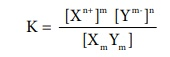Home | | Chemistry 12th Std | Solubility Product

# Solubility Product

We have come across many precipitation reactions in inorganic qualitative analysis.

Solubility Product

We have come across many precipitation reactions in inorganic qualitative analysis. For example, dil HCl is used to precipitate Pb2+ ions as PbCl2 which is sparingly soluble in water. Kidney stones are developed over a period of time due to the precipitation of Ca2+ (as calcium oxalate etcŌĆ”). To understand the precipitation, let us consider the solubility equilibria that exist between the undissociated sparingly soluble salt and its constituent ions in solution.

For a general salt X m Yn ,The equilibrium constant for the above is

K = { [Xn+ ]m [Ym- ]n } / { [Xm Ym ] }In solubility equilibria, the equilibrium constant is referred as solubility product constant (or) Solubility product.

In such heterogeneous equilibria, the concentration of the solid is a constant and is omitted in the above expression

Ksp = [Xn+ ]m [Ym- ]n

The solubility product of a compound is defined as the product of the molar concentration of the constituent ions, each raised to the power of its stoichiometric co ŌĆō efficient in a balanced equilibrium equation.

Solubility product finds useful to decide whether an ionic compound gets precipitated when solution that contains the constituent ions are mixed.

When the product of molar concentration of the constituent ions i.e., ionic product, exceeds the solubility product then the compound gets precipitated.

The expression for the solubility product and the ionic product appears to be the same but in the solubility product expression, the molar concentration represents the equilibrium concentration and in ionic product, the initial concentration (or) concentration at a given time ŌĆśtŌĆÖ is used.

In general we can summarise as,

Ionic product > Ksp , precipitation will occur and the solution is super saturated.

Ionic product < Ksp , no precipitation and the solution is unsaturated.

Ionic product = Ksp , equilibrium exist and the solution is saturated.

### Example 8.9

Indicate find out whether lead chloride gets precipitated or not when 1 mL of 0.1M lead nitrate and 0.5 mL of 0.2 M NaCl solution are mixed? Ksp of PbCl2 is 1.2 ├Ś 10-5.Ionic product = (6.7 ├Ś 10-2 )(6.7 ├Ś10ŌłÆ2 )2 = 3.01 ├Ś 10-4

Since, the ionic product 3.01 ├Ś 10-4 is greater than the solubility product (1.2 ├Ś 10-5 ) , PbCl2 will get precipitated.

## Determination of solubility product from molar solubility

Solubility product can be calculated from the molar solubility i.e., the maximum number of moles of solute that can be dissolved in one litre of the solution.

For a solute Xm Yn ,

Xm Yn (s) Ōåö mXn+ (aq) + nYm-(aq)

From the above stoichiometrically balanced equation we have come to know that 1 mole of Xm Yn (s) dissociated to furnish ŌĆśmŌĆÖ moles of Xn+ and ŌĆśnŌĆÖ moles of Ym- if ŌĆśsŌĆÖ is molar solubility of Xm Yn , then

[Xn+] = ms and [ Ym- ]=ns

Ōł┤Ksp = [Xn+]m [Ym-]n

Ksp = (ms)m (ns)n

Ksp =(m)m (n)n (s)m+n

### Example 8.10

Establish a relationship between the solubility product and molar solubility for the Following

a. BaSO4

b.Ag2 (CrO4 )

BaSO4 (s) ŌåÉH2OŌåÆ Ba2+ (aq) + SO42-(aq)

Ksp =[Ba2+ ][SO42-]

= (s) (s)

Ksp = S2

Ag2 CrO4 (s) ŌåÉH2OŌåÆ 2Ag + (aq) + CrO42-(aq)

Ksp = [Ag+ ]2[CrO42-]

= (2s)2 (s)

Ksp =4s3

Tags : Ionic Equilibrium | Chemistry , 12th Chemistry : UNIT 8 : Ionic Equilibrium
Study Material, Lecturing Notes, Assignment, Reference, Wiki description explanation, brief detail
12th Chemistry : UNIT 8 : Ionic Equilibrium : Solubility Product | Ionic Equilibrium | Chemistry